﻿ 基于K-Means聚类的股票分类研究 Re-search on Stock Classification Based on K-Means Clustering

Statistics and Application
Vol. 08  No. 02 ( 2019 ), Article ID: 29640 , 5 pages
10.12677/SA.2019.82031

Research on Stock Classification Based on K-Means Clustering

Menghan Zhang, Mu Yang, Zai Chen

Hangzhou Dianzi University, Hangzhou Zhejiang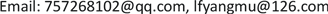Received: Mar. 19th, 2019; accepted: Apr. 3rd, 2019; published: Apr. 10th, 2019ABSTRACT

With the development of China’s economic market and the gradual improvement of the stock market, there are about 2000 stocks in China’s stock market at present, and more and more people regard stocks as a major investment method. We always expect to obtain the maximum benefit with the minimum risk when investing. Facing the huge stock market and complicated stock data, cluster analysis is particularly important for reasonable analysis and selection of stocks. In this paper, we use k-means clustering to cluster 20 randomly selected stocks, then we analyze various types and give corresponding investment suggestions.

Keywords:K-Means Cluster, Stock Analysis, Investment RecommendationsCopyright © 2019 by authors and Hans Publishers Inc.

This work is licensed under the Creative Commons Attribution International License (CC BY).

http://creativecommons.org/licenses/by/4.0/1. 前言

2. 研究目的与方法

2.1. 研究目的

2.2. 研究方法

3. 经典K-means聚类算法

$\underset{j=1}{\overset{m}{\cup }}{C}_{j}=\Omega$ (1)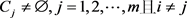(2)

${C}_{i}\cap {C}_{j}=\varnothing ,i,j=1,2,\cdots ,m$ (3)

$J=\underset{j=1}{\overset{m}{\sum }}\underset{i=1}{\overset{{n}_{j}}{\sum }}d\left({x}_{i},{z}_{j}\right),{x}_{i}\in {C}_{j}$ (4)

I：从数据集 $\Omega$ 中随机选择 $m$ 个样本作为初始聚心；

II：根据类 ${C}_{j}\left(j=1,2,\cdots ,m\right)$ 中所含样本的均值，计算每个样本到各类聚心 ${z}_{j}$ 的距离，把其归到距离最小的类；

III：重新计算类 ${C}_{j}\left(j=1,2,\cdots ,m\right)$ 的聚心 ${z}_{j}$

IV：计算本次迭代的聚类质量评估函数 $J\left(t\right)$ 并和上次迭代的聚类质量评估函数 $J\left(t-1\right)$ 比较；若两者之差满足阈值则算法结束，否则转到II继续执行。

4. 数据来源

5. 聚类结果分析Table 1. Classification results of K-means clustering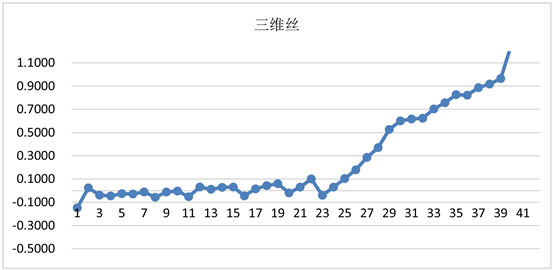Figure 1. Line chart: stock yield of Savings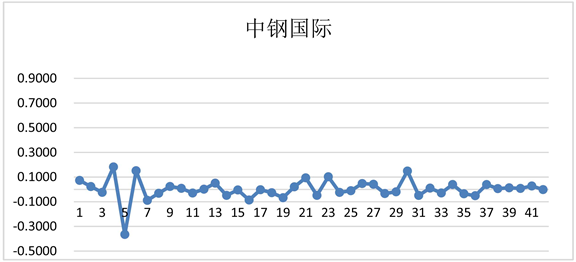Figure 2. Line chart: stock yield of Sinosteel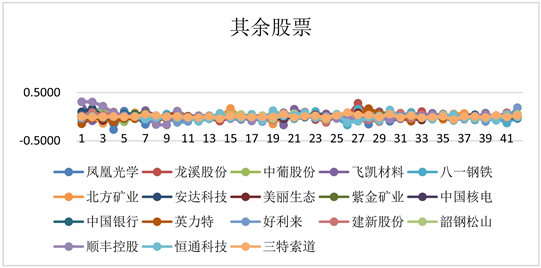Figure 3. Line chart: stock yield of other stocks

6. 结束语

Re-search on Stock Classification Based on K-Means Clustering[J]. 统计学与应用, 2019, 08(02): 277-281. https://doi.org/10.12677/SA.2019.82031

1. 1. 李庆东. 聚类分析在股票分析中的应用[J]. 辽宁石油化工大学学报, 2005, 25(3): 94-96.

2. 2. 李慧. 聚类分析在股票投资分析中的应用[J]. 商, 2015(27): 199.

3. 3. 吴曼琪. 基于K均值聚类的ST股票分类研究及投资策略[J]. 中国城市经济, 2010(8X): 26-26.

4. 4. 洪月华. 蜂群K-means聚类算法改进研究[J]. 科技通报, 2016, 32(4): 170-173.

5. 5. 王强. 聚类分析模型在股票市场的应用[J]. 经济界, 2016(5): 101.

6. 6. 邓攀. 湖南省工业企业资本效率的实证研究[D]: [硕士学位论文]. 长沙: 中南大学, 2006.

7. 7. 王标. “乞丐帮主”的ST股操作全攻略[J]. 私人理财, 2005(4): 66-68.